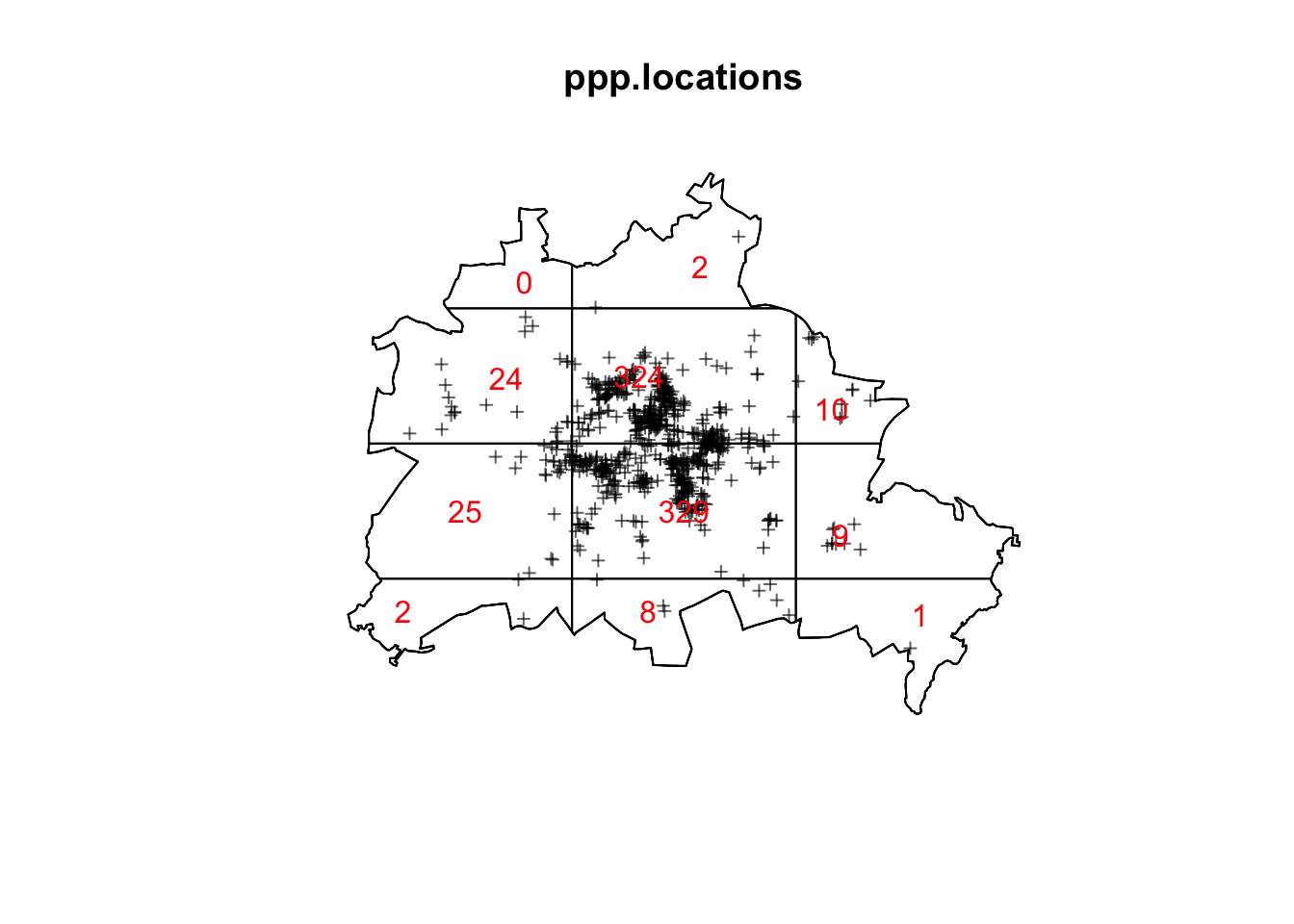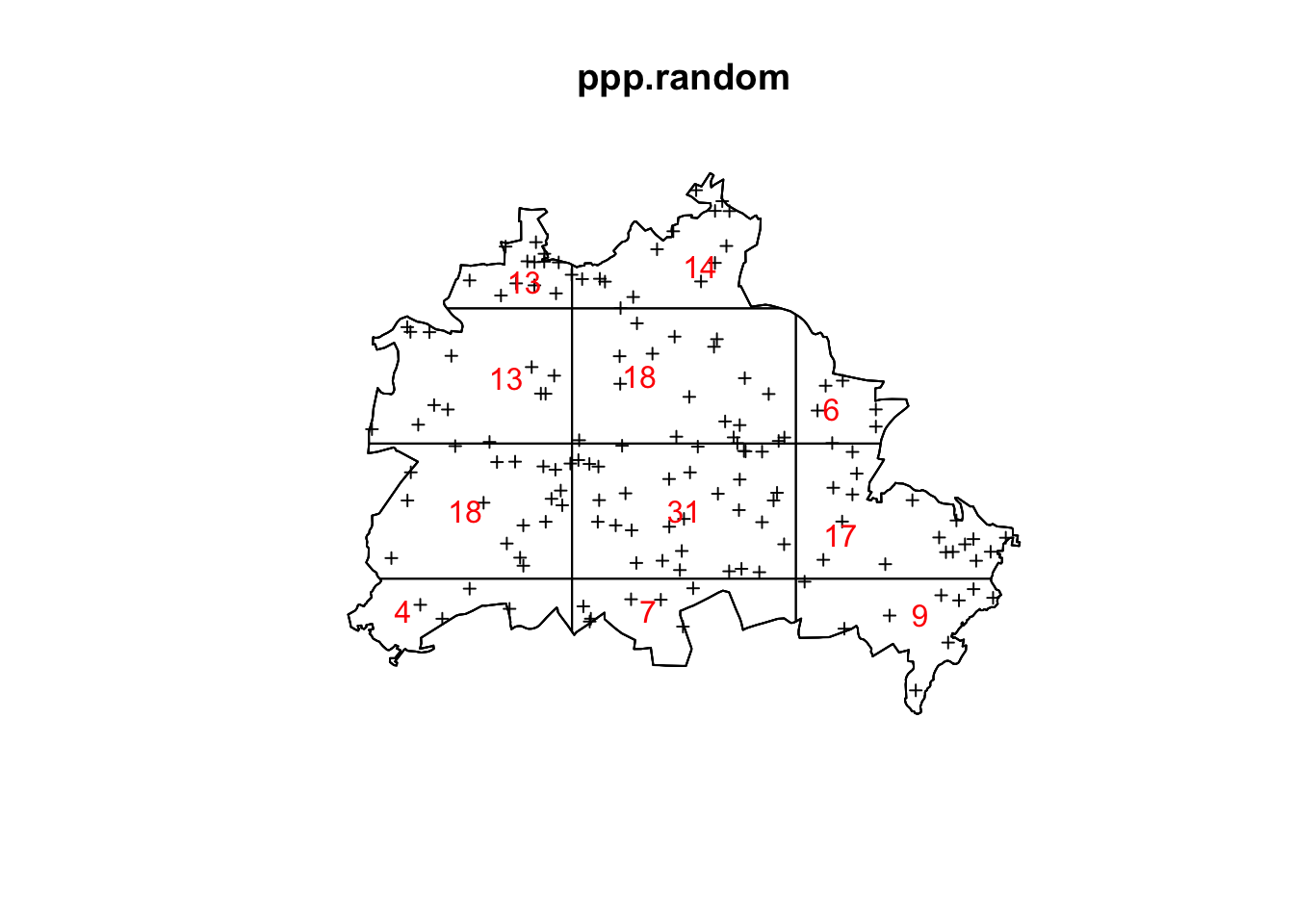Intensity is the average density of points or in other words the expected number of points per unit area. The intensity may be constant (uniform) or may vary from location to location (non-uniform or inhomogeneous).

One approach to evaluate the intensity is to divide the study area into quadrats, and count the numbers of points falling into each quadrat. If the points have uniform intensity, and are completely random, then the quadrat counts should be Poisson random numbers with constant mean. We may test that hypothesis by using the $$\chi^2$$ goodness-of-fit test statistic

$\chi^2 = \sum\frac{(\text{observed}-\text{expected})^2}{\text{expected}}$

In R we make use of the quadratcount() and quadrat.test() functions of the spatstat package. First, we load the Berlin city data sets we created in the previous section.

load(url("https://userpage.fu-berlin.de/soga/300/30100_data_sets/ppp_data_berlin.RData"))
load(url("https://userpage.fu-berlin.de/soga/300/30100_data_sets/berlin_district.RData"))
library(spatstat)
plot(ppp.locations, pch=3, cex=0.6)
plot(qc.loc, add=T, textargs = list(col='red'))quadrat.test(qc.loc)
##
##  Chi-squared test of CSR using quadrat counts
##  Pearson X2 statistic
##
## data:
## X2 = 1150.1, df = 10, p-value < 2.2e-16
## alternative hypothesis: two.sided
##
## Quadrats: 11 tiles (irregular windows)

The very low p-value indicates that the intensity is non-constant. Such an point process is referred to as IPP and its intensity can be estimated non-parametrically, e.g., with kernel smoothing (Bivand et al. 2008).

Let us repeat this approach for the ppp.random data set.

qc.rand <- quadratcount(ppp.random, nx=3, ny=4)
plot(ppp.random, pch=3, cex=0.6)
plot(qc.rand, add=T, textargs = list(col='red'))quadrat.test(qc.rand)
##
##  Chi-squared test of CSR using quadrat counts
##  Pearson X2 statistic
##
## data:
## X2 = 19.377, df = 10, p-value = 0.07145
## alternative hypothesis: two.sided
##
## Quadrats: 11 tiles (irregular windows)

The high p-value $$(p > 0.05)$$ indicates a HPP, where the intensity is a constant $$\lambda(u) = \lambda = \frac{n}{\vert A \vert}$$, with $$n$$ being the number of points observed in region $$A$$, and $$\vert A \vert$$ being the area of region $$A$$.

#### Kernel density estimate

There are different ways to estimate the intensity for an IPP. One of them is a non-parametrically estimation by means of kernel smoothing. If we observe $$n$$ points $$\{x_i\}_{i=1}^n$$ then the form of a kernel smoothing estimator is

$\hat{\lambda}(x) = \frac{1}{h^2}\sum_{i=1}^n\frac{\kappa(\frac{\Vert x-x_i\Vert}{h})}{q(\Vert x \Vert)}\text{,}$

where $$x_i \in \{x_1, x_2,..., x_n\}$$ is an observed point, $$h$$ is the bandwidth, $$q(\Vert x \Vert)$$ is a border correction to compensate for observations missing due to edge effects, and $$\kappa(u)$$ is a bivariate and symmetrical kernel function.

R currently implements a two-dimensional quartic kernel function:

$\kappa(u) = \begin{cases} \frac{3}{\pi}(1-\Vert u \Vert^2)^2 & \text{if } u \in (-1, 1) \\ 0 & \text{otherwise} \end{cases}$

where $$\Vert u \Vert^2=u_1^2+u_2^2$$ is the squared norm of point $$u=(u_1, u_2)$$.

There is no general rule for selecting the bandwidth $$h$$, which governs the level of smoothing. Small bandwidths result in spikier maps and large bandwidths result in smoother map. It is reasonable to use several values depending on the process under consideration, and choose a value that seems plausible (Bivand et al. 2008).

#### Kernel density plot (2-D)

A kernel density plot is the graphical representation of kernel smoothing. In R and contributed packages there are several functions available for the visualization of kernel density plots. Here we use the density.ppp() function from the spatstat package.

The density.ppp() provides an estimate of the intensity function of the point process that generated the point pattern data. The argument sigma is taken as the standard deviation of the isotropic Gaussian kernel, corresponding to the bandwidth parameter. A small standard deviation results in a spikier density plot and a large standard deviation results in a smoother density plot.

We code a loop to apply the density.ppp() function on our three data sets, ppp.locations, ppp.regular, and ppp.random with tree different values for sigma $$(1000, 2500, 3000)$$.

par(mfrow=c(3,3), mar=c(0,0,1,2))
sigma <- c(1000, 2500, 5000)
data <- list(ppp.locations, ppp.regular, ppp.random)
main <- c('Locations', 'Regular', 'Random')
for (i in 1:3){
for (j in 1:3){
ds <- density.ppp(data[[i]], sigma=sigma[j])
plot(ds,
main = paste0(main[i], ', sigma: ', sigma[j]))
}